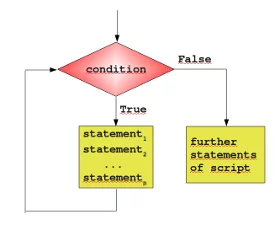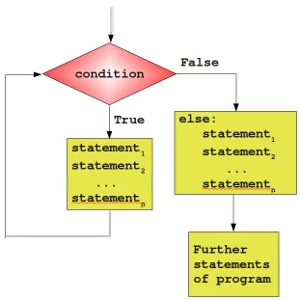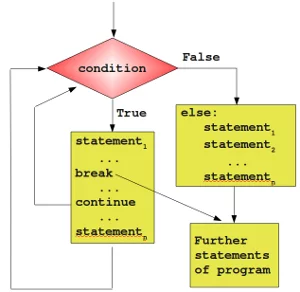# 19. While Loops

## General Structure of a LoopIn this chapter of our python course, we delve into the powerful world of while loops, a fundamental concept in programming. You will hardly find any programming language with a way to loop over code. While loops provide a mechanism for executing a block of code (or sequence of statements) repeatedly as long as a certain condition holds true. The code within the loop, i.e. the code carried out repeatedly, is called the body of the loop.

Essentially, there are three different kinds of loops:

• Count-controlled loops A construction for repeating a loop a certain number of times. An example of this kind of loop is the for-loop of the programming language C:

for (i=0; i <= n; i++)

Python doesn't have this kind of loop.

• Condition-controlled loop A loop will be repeated until a given condition changes, i.e. changes from True to False or from False to True, depending on the kind of loop. There are 'while loops' and 'do while' loops with this behaviour.

• Collection-controlled loop This is a special construct which allows looping through the elements of a 'collection', which can be an array, list or other ordered sequence. Like the for loop of the bash shell (e.g. for i in *, do echo $i; done) or the foreach loop of Perl.Python supplies two different kinds of loops: the while loop and the for loop, which correspond to the condition-controlled loop and collection-controlled loop. Most loops contain a counter or more generally, variables, which change their values in the course of calculation. These variables have to be initialized before the loop is started. The counter or other variables, which can be altered in the body of the loop, are contained in the condition. Before the body of the loop is executed, the condition is evaluated. If it evaluates to False, the while loop is finished. In other words, the program flow will continue with the first statement after the while statement, i.e. on the same indentation level as the while loop. If the condition is evaluated to True, the body, - the indented block below the line with "while" - gets executed. After the body is finished, the condition will be evaluated again. The body of the loop will be executed as long as the condition yields True. Live Python training Enjoying this page? We offer live Python training courses covering the content of this site. Enrol here ## A Simple Example with a While Loop It's best to illustrate the operating principle of a loop with a simple Python example. The following small script calculates the sum of the numbers from 1 to 100. We will later introduce a more elegant way to do it. n = 100 total_sum = 0 counter = 1 while counter <= n: total_sum += counter counter += 1 print("Sum of 1 until " + str(n) + " results in " + str(total_sum))  ### OUTPUT: Sum of 1 until 100 results in 5050  ## Exercise Write a program, which asks for the initial balance K0 and for the interest rate. The program shall calculate the new capital K1 after one year including the interest. Extend the program with a while-loop, so that the capital Kn after a period of n years can be calculated. K = float(input("Starting capital? ")) p = float(input("Interest rate? ")) n = int(input("Number of years? ")) i = 0 while i < n: K += K * p / 100.0 # or K *= 1 + p / 100.0 i += 1 print(i, K) print("Capital after " + str(n) + " ys: " + str(K))  ### OUTPUT: 1 1023.0 2 1046.529 3 1070.599167 4 1095.2229478410002 5 1120.413075641343 6 1146.182576381094 7 1172.544775637859 8 1199.5133054775297 9 1227.1021115035128 10 1255.3254600680937 11 1284.19794564966 12 1313.734498399602 13 1343.950391862793 14 1374.861250875637 15 1406.4830596457768 16 1438.8321700176298 17 1471.9253099280352 18 1505.77959205638 19 1540.4125226736767 20 1575.8420106951712 Capital after 20 ys: 1575.8420106951712  Live Python training Enjoying this page? We offer live Python training courses covering the content of this site. Upcoming online Courses Enrol here ## The else PartSimilar to the if statement, the while loop of Python has also an optional else part. This is an unfamiliar construct for many programmers of traditional programming languages. The statements in the else part are executed, when the condition is not fulfilled anymore. Some might ask themselves now, where the possible benefit of this extra branch is. If the statements of the additional else part were placed right after the while loop without an else, they would have been executed anyway, wouldn't they? We need to understand a new language construction, i.e. the break statement, to obtain a benefit from the additional else branch of the while loop. The general syntax of a while loop looks like this: while condition: statement_1 ... statement_n else: statement_1 ... statement_n   while potatoes: peel cut else: pot pot on oven cook for 40 minutes wash salad and so on  ## Premature Termination of a while LoopSo far, a while loop only ends, if the condition in the loop head is fulfilled. With the help of a break statement a while loop can be left prematurely, i.e. as soon as the control flow of the program comes to a break inside of a while loop (or other loops) the loop will be immediately left. "break" shouldn't be confused with the continue statement. "continue" stops the current iteration of the loop and starts the next iteration by checking the condition. Here comes the crucial point: If a loop is left by break, the else part is not executed. This behaviour will be illustrated in the following example, a little guessing number game. A human player has to guess a number between a range of 1 to n. The player inputs his guess. The program informs the player, if this number is larger, smaller or equal to the secret number, i.e. the number which the program has randomly created. If the player wants to gives up, he or she can input a 0 or a negative number. Hint: The program needs to create a random number. Therefore it's necessary to include the module "random". import random upper_bound = 20 lower_bound = 1 #to_be_guessed = int(n * random.random()) + 1 to_be_guessed = random.randint(lower_bound, upper_bound) guess = 0 while guess != to_be_guessed: guess = int(input("New number: ")) if guess > 0: if guess > to_be_guessed: print("Number too large") elif guess < to_be_guessed: print("Number too small") else: # 0 means giving up print("Sorry that you're giving up!") break # break out of a loop, don't execute "else" else: print("Congratulations. You made it!")  ### OUTPUT: Number too large Congratulations. You made it!  The previous program doesn't check if the number makes sense, i.e. if the number is between the boundaries upper_bound and over_bound. We can improve our program. The boundaries have to be adapted according to the user input: import random upper_bound = 20 lower_bound = 1 to_be_guessed = random.randint(lower_bound, upper_bound) guess = 0 while guess != to_be_guessed: guess = int(input("New number: ")) if guess == 0: # giving up print("Sorry that you're giving up!") break # break out of a loop, don't execute "else" if guess < lower_bound or guess > upper_bound: print("guess not within boundaries!") elif guess > to_be_guessed: upper_bound = guess - 1 print("Number too large") elif guess < to_be_guessed: lower_bound = guess + 1 print("Number too small") else: print("Congratulations. You made it!")  ### OUTPUT: Number too large guess not within boundaries! Number too small guess not within boundaries! guess not within boundaries! Number too large Congratulations. You made it!  Live Python training Enjoying this page? We offer live Python training courses covering the content of this site. Enrol here ## Exercises ### Exercise 1: Dog Age to Human Age We had a program to convert a dog age to a human age in our chapter Conditional Statements. This was the program: dog_age = int(input("Age of the dog: ")) print() if dog_age <= 0: human_age = -1 elif dog_age == 1: human_age = 14 else: # this means: dog_age >= 2: human_age = 22 + (dog_age - 2) * 5 if human_age > 0: print("Corresponds to " + str(human_age) + " human years!") else: print("Negative values or zero makes no sense for a dog age!")  ### OUTPUT: Corresponds to 27 human years!  Write a version with a while loop so that people can keep on converting dog ages. 0 or a negative value means that they want to finish. ### Exercise 2: Factorial Calculator Write a Python program that calculates the factorial of a number entered by the user using a while loop. The factorial of a non-negative integer n, denoted in mathematics as n!, is the product of all positive integers from 1 to n. For example, 5! (read as "5 factorial") is equal to 5 * 4 * 3 * 2 * 1, which is 120. ### Exercise 3: Password Checker Write a Python program that simulates a simple password checker. The program should ask the user to enter a password and continue to prompt them until they enter the correct password. Once the correct password is entered, the program should print a success message. ### Exercise 4: Pawword Checker Continued Let's extend the previous password checker example by adding a maximum number of attempts. If the user exceeds the maximum number of attempts, the program should lock them out. ### Exercise 5: Prime Number Checker Write a Python program that checks whether a given positive integer is a prime number. The program should ask the user to input a number and then use a while loop to determine if the number is prime. ### Exercise 6: Fibonacci Sequence Write a Python program that generates the Fibonacci sequence up to a specified number of terms. The Fibonacci sequence is a series of numbers where each number is the sum of the two preceding ones. The first two numbers in the sequence are typically 0 and 1. ### Exercise 7: The Collatz conjecture is one of the most famous unsolved problems in mathematics. The conjecture asks whether repeating two simple arithmetic operations will eventually transform every positive integer into 1. It concerns sequences of integers in which each term is obtained from the previous term as follows: if the previous term is even, the next term is one half of the previous term. If the previous term is odd, the next term is 3 times the previous term plus 1. The conjecture is that these sequences always reach 1, no matter which positive integer is chosen to start the sequence. A mathematical formultation if the Collatz conjecture: The Collatz conjecture states that for any positive integer$n$, the sequence$\{a_k\}$will eventually reach the number$1$for some$k$, if$a_0$is set to$n$} $$a_{k+1} = \begin{cases} \frac{a_k}{2} & \text{if a_k is even} \\ 3a_k + 1 & \text{if a_k is odd} \end{cases}$$ • Write a program to print out the sequence of a number$n\$
• How long is the sequence for the number 271114753?
• Write a program to print the lengths of the Collatz sequences for the numbers from 1 to 100.

## Solutions

### Solution to Exercise 1:

dog_age = 1
while dog_age > 0:
dog_age = int(input("Age of the dog: (0 or negative number to finish)"))
print()
if dog_age <= 0:
human_age = -1
elif dog_age == 1:
human_age = 14
elif dog_age >= 2:
human_age = 22 + (dog_age - 2) * 5

print("Corresponds to  " + str(human_age) + " human years!")

print("Program finished!")


### OUTPUT:

Corresponds to  27 human years!

Corresponds to  22 human years!

Corresponds to  -1 human years!
Program finished!


The next version uses the assignment operator (walrus operator):

while (dog_age := int(input("Age of the dog: (0 or negative number to finish)"))) > 0:
print()
if dog_age <= 0:
human_age = -1
elif dog_age == 1:
human_age = 14
elif dog_age >= 2:
human_age = 22 + (dog_age - 2) * 5

print("Corresponds to " + str(human_age) + " human years!")

print("Program finished!")


### OUTPUT:

Corresponds to 27 human years!

Corresponds to 22 human years!
Program finished!


### Solution to Exercise 2:

n = int(input("Enter a positive integer: "))

factorial = 1
counter = 1

while counter <= n:
factorial *= counter
counter += 1

print(f"{n}! = {factorial}")


### OUTPUT:

30! = 265252859812191058636308480000000


### Solution to Exercise 3:

correct_password = "password123"

user_input = input("Enter the password: ")

# Use a while loop to repeatedly prompt for the password until it's correct
user_input = input("Enter the password: ")

# Display a success message once the correct password is entered
print("Success! You've entered the correct password.")


### OUTPUT:

Incorrect password. Please try again.
Success! You've entered the correct password.


### Solution to Exercise 4:

correct_password = "password123"
max_attempts = 3
attempts = 0

while attempts < max_attempts:
user_input = input("Enter the password: ")

print("Success! You've entered the correct password.")
break
else:
attempts += 1
remaining_attempts = max_attempts - attempts
if remaining_attempts > 0:
print(f"Incorrect password. You have {remaining_attempts} attempt(s) remaining.")
else:
print("Maximum attempts exceeded. You are now locked out.")


### OUTPUT:

Incorrect password. You have 2 attempt(s) remaining.
Incorrect password. You have 1 attempt(s) remaining.
Maximum attempts exceeded. You are now locked out.


### Solution to Exercise 5:

number = int(input("Enter a positive integer: "))

# Initialize a variable to keep track of the divisors
divisor = 1

# Initialize a variable to determine if the number is prime
is_prime = True

# Use a while loop to check for divisors
while divisor <= number // 2:
divisor += 1
if number % divisor == 0 and is_prime:
is_prime = False
break  # If a divisor is found, exit the loop

# Display the result
if is_prime:
print(number, "is a prime number.")
else:
print(number, "is not a prime number.")


### OUTPUT:

11 is a prime number.


### Solution to Exercise 6:

n_terms = int(input("Enter the number of terms for the Fibonacci sequence: "))

# Initialize variables for the first two terms, the start of the sequence
old, new = 0, 1

count = 0
while count < n_terms:
print(old, end=" ")  # Print the current term
old, new = new, old + new  # Update term1 and term2
count += 1

print()


### OUTPUT:

0 1 1 2 3 5 8 13 21 34 55 89 144 233 377 610 987 1597 2584 4181


### Solution to Exercise 7:

The algorithm for the Collatz sequene works like this:

• If n is even, divide it by 2.
• If n is odd, multiply it by 3 and add 1.
• Repeat the process with the calculated value as the new value of n, and continue until n becomes 1
n = int(input("Enter a positive integer: "))

if n <= 0:
else:
print(f"Collatz sequence for {n}:")
while n != 1:
if n % 2 == 0:
n = n // 2
else:
n = 3 * n + 1
print(n)


### OUTPUT:

Collatz sequence for 11:
34
17
52
26
13
40
20
10
5
16
8
4
2
1

n = int(input("Enter a positive integer: "))
length_of_sequence = 0
if n <= 0:
else:
while n != 1:
if n % 2 == 0:
n = n // 2
else:
n = 3 * n + 1
length_of_sequence += 1
print(f"Length of sequence: {length_of_sequence}")


### OUTPUT:

Length of sequence: 279


Program to print the lengths of the Collatz sequences for the numbers from 1 to 100.

counter = 1
stop_value = 100
while counter <= stop_value:
#print(f"Collatz sequence for {counter}:")
n = counter
length_of_sequence = 0
while n != 1:
if n % 2 == 0:
n = n // 2
else:
n = 3 * n + 1
#print(n, end=", ")
length_of_sequence += 1
print(f"{counter}: {length_of_sequence}", end=", ")
counter += 1
print()


### OUTPUT:

1: 0, 2: 1, 3: 7, 4: 2, 5: 5, 6: 8, 7: 16, 8: 3, 9: 19, 10: 6, 11: 14, 12: 9, 13: 9, 14: 17, 15: 17, 16: 4, 17: 12, 18: 20, 19: 20, 20: 7, 21: 7, 22: 15, 23: 15, 24: 10, 25: 23, 26: 10, 27: 111, 28: 18, 29: 18, 30: 18, 31: 106, 32: 5, 33: 26, 34: 13, 35: 13, 36: 21, 37: 21, 38: 21, 39: 34, 40: 8, 41: 109, 42: 8, 43: 29, 44: 16, 45: 16, 46: 16, 47: 104, 48: 11, 49: 24, 50: 24, 51: 24, 52: 11, 53: 11, 54: 112, 55: 112, 56: 19, 57: 32, 58: 19, 59: 32, 60: 19, 61: 19, 62: 107, 63: 107, 64: 6, 65: 27, 66: 27, 67: 27, 68: 14, 69: 14, 70: 14, 71: 102, 72: 22, 73: 115, 74: 22, 75: 14, 76: 22, 77: 22, 78: 35, 79: 35, 80: 9, 81: 22, 82: 110, 83: 110, 84: 9, 85: 9, 86: 30, 87: 30, 88: 17, 89: 30, 90: 17, 91: 92, 92: 17, 93: 17, 94: 105, 95: 105, 96: 12, 97: 118, 98: 25, 99: 25, 100: 25,


Live Python training

Enjoying this page? We offer live Python training courses covering the content of this site.

Upcoming online Courses

Enrol here